# OECD Business confidence Index(BCI) data analysis 4 - Time-Series Regression using R - static modelPhoto by Elena Louca on Unsplash

This post is following of above post.

Let's do time-series regression.
Firstly, let's make a static time series model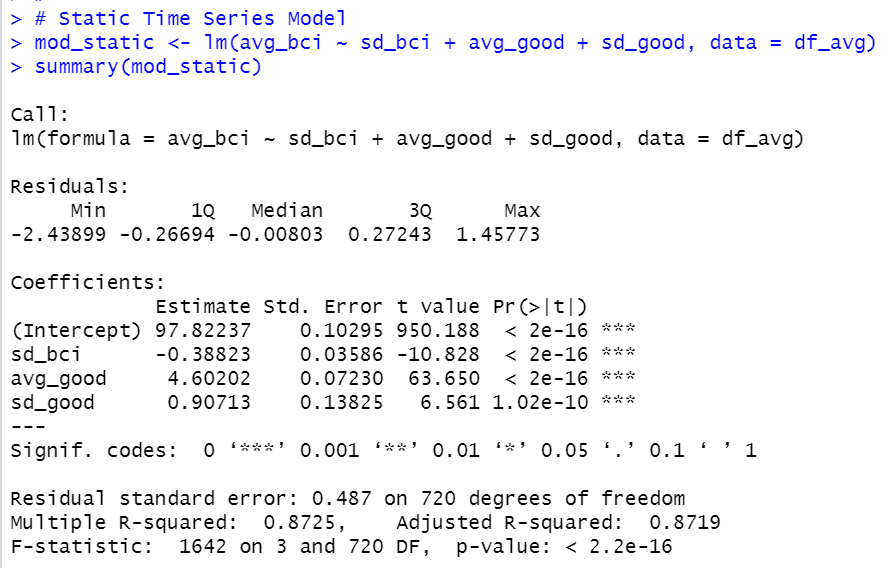In time-series regression, we have to care about serial correlation of the error term.

Firstly, I load dynlm package and lmtest package.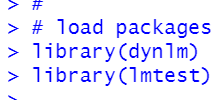Before testing serial correlation, let's plot error term.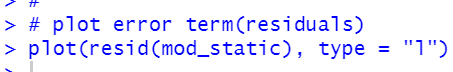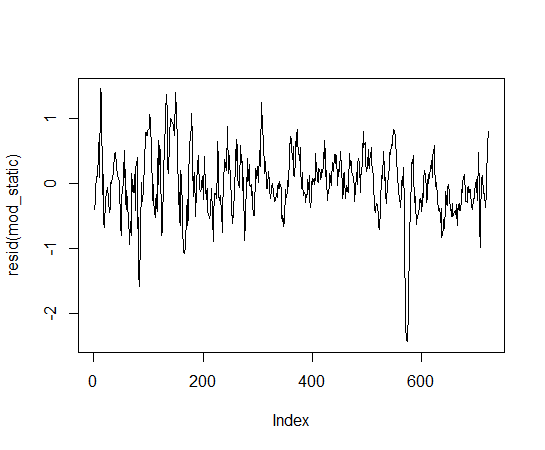Since I don't have good experiences, I cannot tell if there is serial correlation or not by just seeing the plot.

Let's test AR(1) Serial Correlation.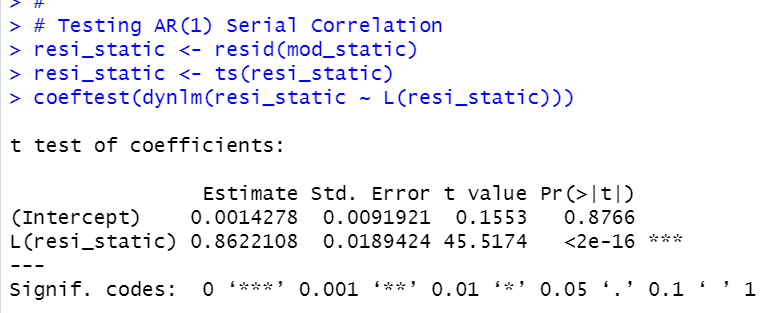p-value is less than 2e-16, it is alomost 0. So mod_static has serial correlation.

We need orcutt package to do Cochrane-Orcutt estimation and I will use cochrane.orcutt() function.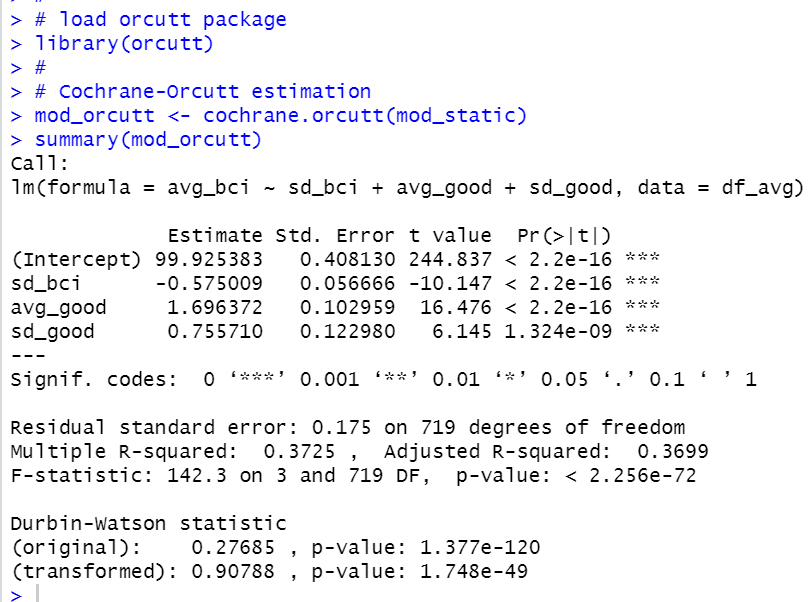Let's compare mod_static and mod_orcutt.
I use stargazer package.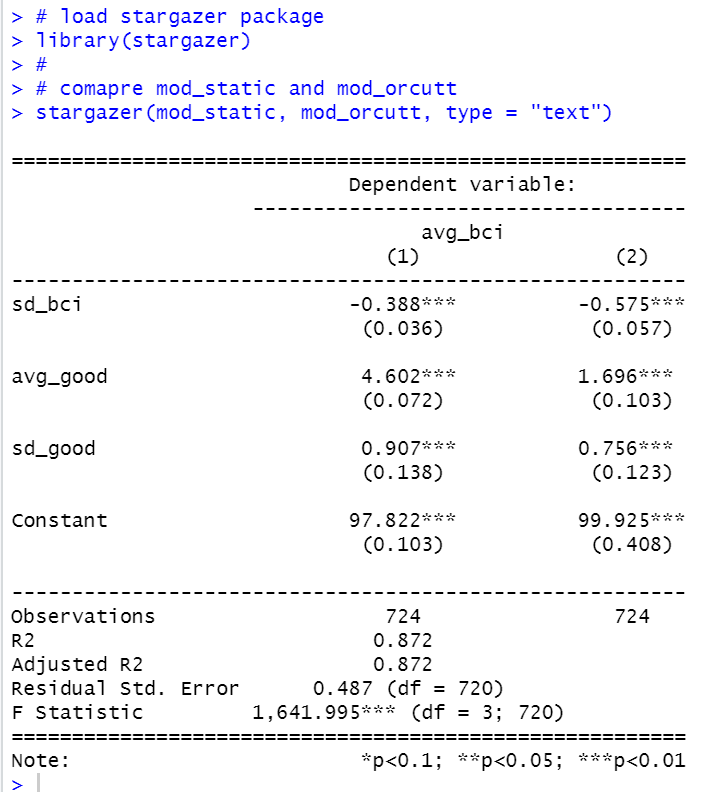sd_bci coefficient is negative and statistically significant. It means that when there are large variance of bci, bci level is lower than when varicance is small.
That's it. Thank you!

Next post is

To see the 1st post,